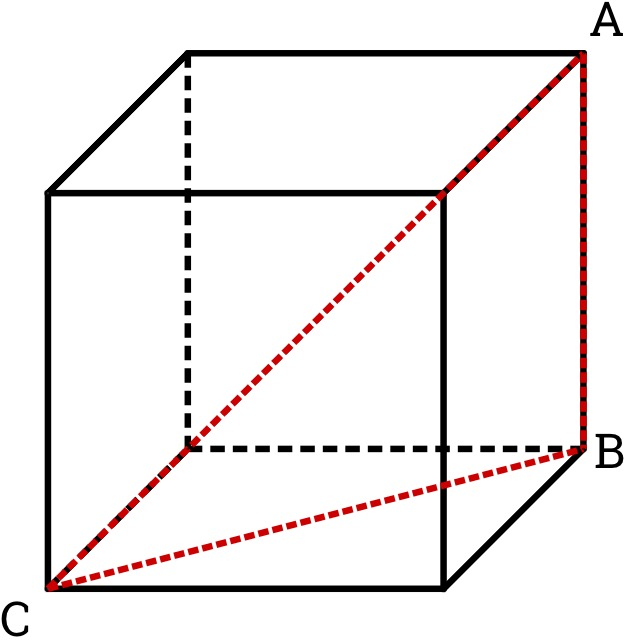# Cubing Makes Perfect

Geometry Level 2A Triangle $ABC$ was formed inside a cube as shown in the picture above. If $AC$ is a space diagonal and $CB$ is a face diagonal, find the measure of $\angle ACB$ in degrees.